# Compare Two Columns In Excel Using VLOOKUPArticle byExcelMojo Team## What Is VLOOKUP To Compare Two Columns In Excel & Find Matches?

The option to compare two columns in Excel using VLOOKUP enables one to find the matches between two columns of data. And a match indicates a data point from one column appears in the other.

Users can compare two columns in Excel using VLOOKUP to find duplicates and similarities between massive lists required to make decisions in Data Analytics.

For example, the table below shows the eligible candidates for Level I and II Tests at a school in columns A and C.

The requirement is to determine the Level I Test eligible candidates who are also eligible for the Level II Test and display the output in column E.

Then, we can compare two columns in Excel using VLOOKUP formula in the target cells and achieve the required output.

In the above, compare two columns in Excel using VLOOKUP formula example, the Excel VLOOKUP function accepts the column A cell value as the lookup value. It checks the name specified in the column A cell, in the given lookup range C2:C8 containing the Level II Test eligible candidates. And as we require the value corresponding to the lookup value in the same row as the match in the lookup range C2:C8 from the same range, the third argument is 1. Next, as the match must be exact, the last argument is 0.

Thus, if we execute the function in a target cell and it finds a match for the specific Level I Test eligible candidate from column A in the Level II Test eligible candidates list in column C. Then, the function output in the target cell will be the Level II Test-eligible candidate name. Otherwise, the function output will be the #N/A error.

###### Key Takeaways
• The compare two columns in Excel using VLOOKUP helps us compare two columns of data for matches. And the function output can be the value corresponding to the lookup value matched in the lookup range or the #N/A error value. While a match indicates a value from one column is present in the other column, the error value #N/A indicates the concerned value in one column is not present in the other.
• Users can compare two Excel columns using the VLOOKUP() to find exact or approximate matches between two large lists in financial modeling.
• The VLOOKUP() accepts three mandatory arguments and one optional argument. While the lookup_value argument will be the lookup value from one column, the table_array argument will be the data range in the other column. And the col_index_num argument will be 1. On the other hand, the last argument, range_lookup, will be TRUE (or 1) or FALSE (or 0) based on whether we require the VLOOKUP() to perform an approximate or exact match.
• We can use Wildcard characters, ‘*’ and ‘?’, to compare two Excel columns using the VLOOKUP() based on a partial match.

### How To Compare Two Columns In Excel Using VLOOKUP (Find Matches)?

The steps to compare two columns in Excel using VLOOKUP to find matches are as follows:

1. First, ensure the data in the two columns to compare are accurate and valid, without unwanted leading and trailing spaces.
2. Please choose the first cell of the target column, where we need to display the columns comparison.
3. Type =VLOOKUP( in the cell. [ Alternatively, type =V or =VL and double-click the VLOOKUP function from the Excel suggestions.]
4. The function accepts four argument values, lookup_value, table_array, col_index_num, and range_lookup. So, enter the function argument values and close the bracket. In this scenario, the lookup_value will be a value from one column, for which we must find a match in the table_array, which will be the other column range. And as the table_array contains only one column, the col_index_num will be 1. Finally, we typically aim to find an exact match for the specified lookup value. Hence, the last argument, range_lookup, will be False (0).
5. Press Enter to execute the VLOOKUP().
6. Copy the formula or iterate steps 2 to 5 in the remaining target cells to view the output for the two columns comparison for matches.

Furthermore, we can compare two columns in Excel using VLOOKUP and highlight differences.

The differences in the two columns comparison indicate those values, which are present in only one of the two columns. And in such scenarios, the VLOOKUP() will return the #N/A error value in the corresponding target cells.

So, we can use the VLOOKUP() within the Excel IFERROR function. The formula will enable us to display a customized comment instead of the error value, which we can use as a medium to highlight the differences between the two given columns.

Alternatively, we can use the VLOOKUP() in the Excel Conditional Formatting feature rule to compare two columns in Excel using VLOOKUP and highlight differences. It can be a way to highlight the error values in the target cells, which indicate the unique lookup values present in only one of the two columns. Otherwise, we can highlight the unique values in the source column containing the lookup values.

#### Basic Example

The below image shows two lists of the top 10 US states by land area and population in columns A and C.

And the requirement is to determine which states from column A are present in column C and display the output in column E.

Then, we can compare two columns in Excel using VLOOKUP method in the target cells to obtain the desired output.

1. Choose cell E2 and enter the VLOOKUP() as shown below.

=VLOOKUP(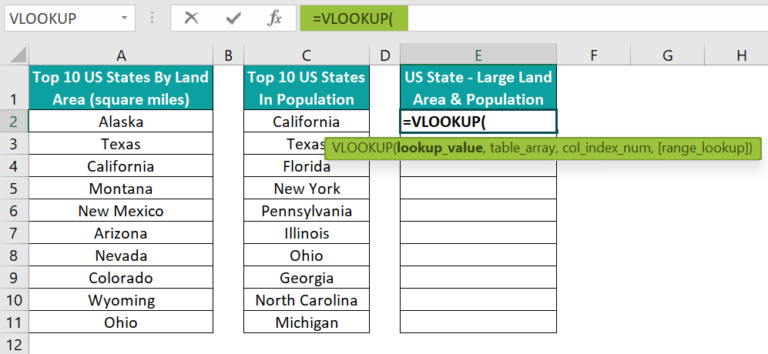Next, enter the cell reference A2 from the first column as the lookup_value argument value and a comma.
=VLOOKUP(A2,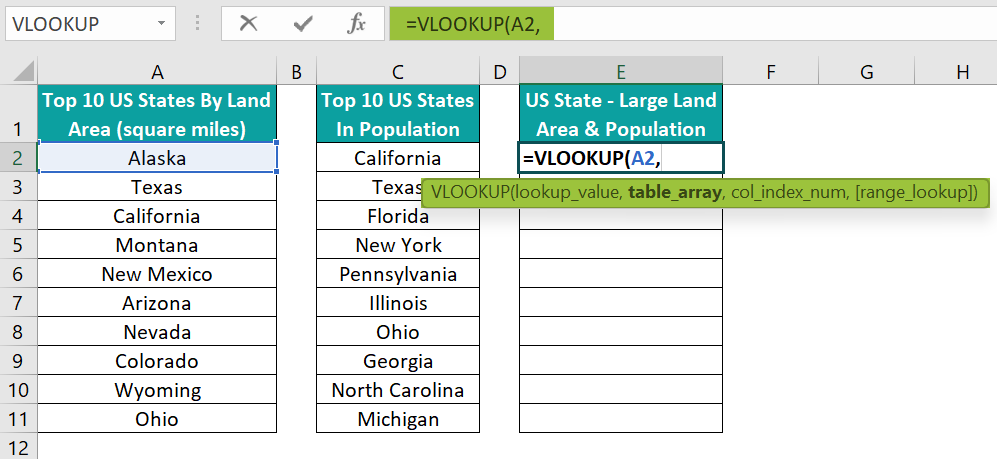And then, enter the cell range \$C\$2:\$C\$11 from the second column as the table_array, where we must search the lookup value for a match, followed by a comma.

Please note that we use the absolute reference in Excel for the table_array range, as we will copy the VLOOKUP() formula in the remaining target cells in column E.

And the table_array range should be constant in the VLOOKUP() in all the target cells.
=VLOOKUP(A2, \$C\$2:\$C\$11,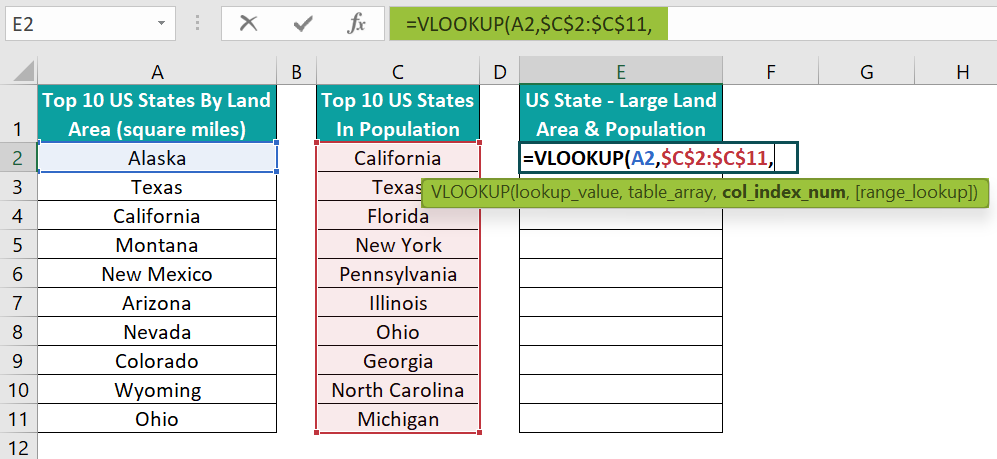Next, as the table_array has only one column, the col_index_num argument value will be 1. And once we enter a comma, Excel will show the options to choose the last argument, range_lookup, value for an approximate or exact match.
=VLOOKUP(A2, \$C\$2:\$C\$11,1,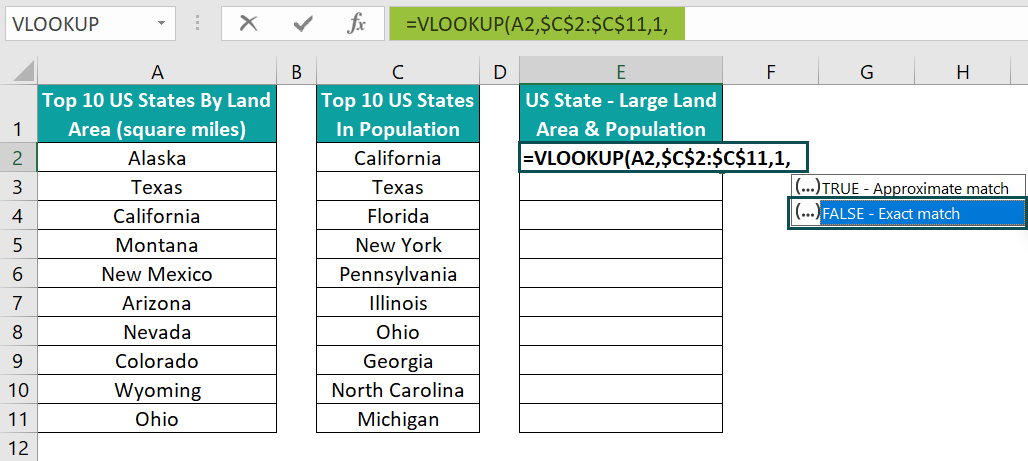Double-click on FALSE to select the last argument value for an exact match.
=VLOOKUP(A2, \$C\$2:\$C\$11,1,FALSE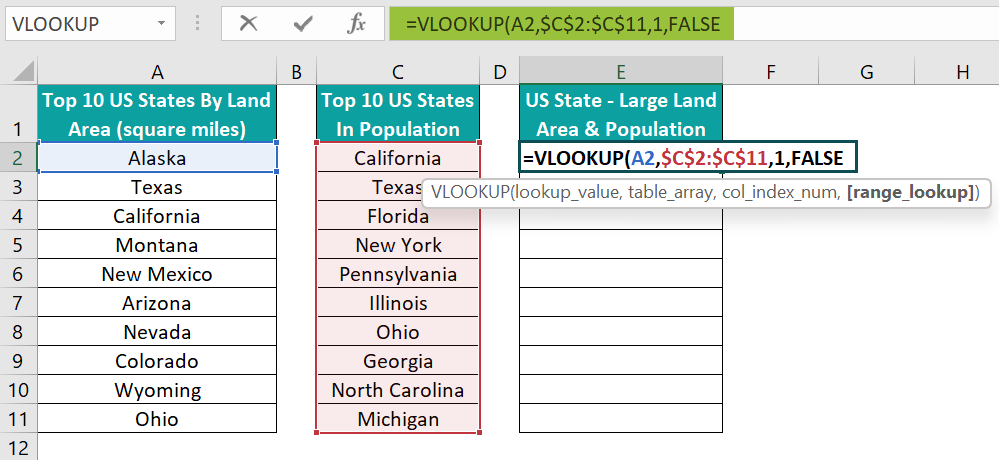Finally, close the bracket.
=VLOOKUP(A2, \$C\$2:\$C\$11,1,FALSE)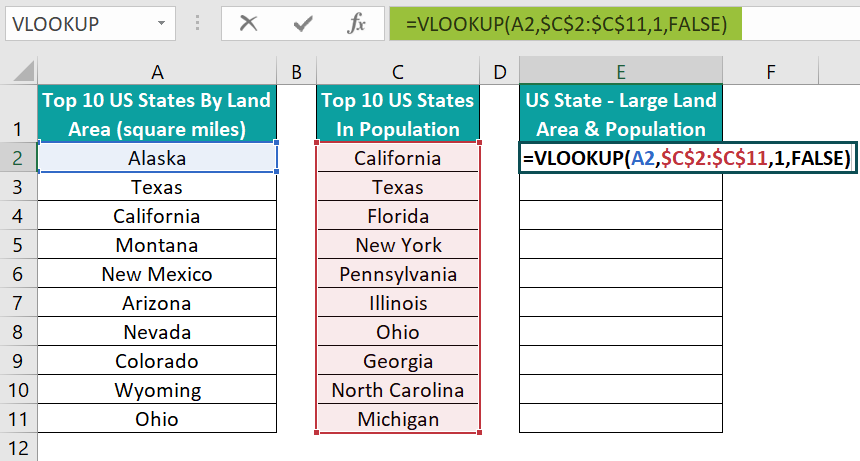2. Press Enter to execute the VLOOKUP() in the target cell.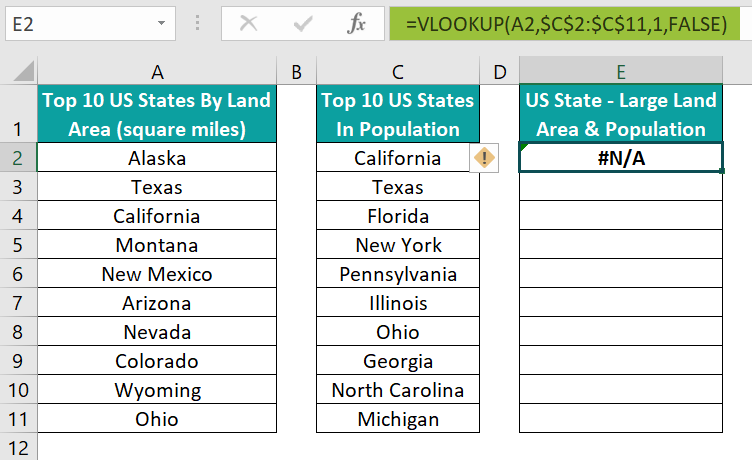3. Using the Excel fill handle, update the formula in the remaining target cells E3:E11.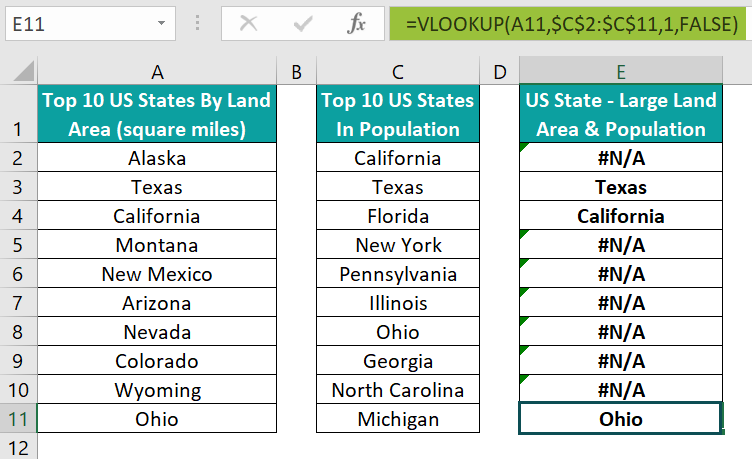Let us check the cell E11 formula to know how it executes.

The VLOOKUP() looks up the cell A11 value, Ohio, in the lookup range C2:C11. And the value matches the cell C8 data, and we need the value corresponding to the lookup value in the same row as the match in the cell range C2:C11. So, the function returns the value Ohio as the output.

Thus, the outcome shows that three states from column A, Texas, California, and Ohio, are in column C. And the remaining values in column A are unique, which the error value #N/A in the corresponding rows of column E represents.

Furthermore, let us see how to highlight the differences between columns A and C. And here, let us highlight the values present in column A and not in column C and the error values in column E, which also denote the differences.

4. Select the cell range A2:A11 and E2:E11 and choose the Home tab → Conditional Formatting drop-down.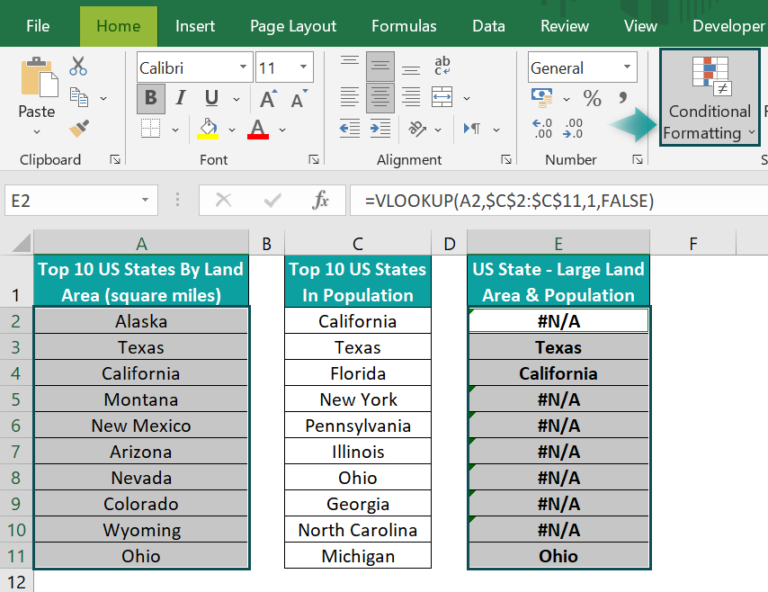And choose the New Rule option from the Conditional Formatting drop-down list.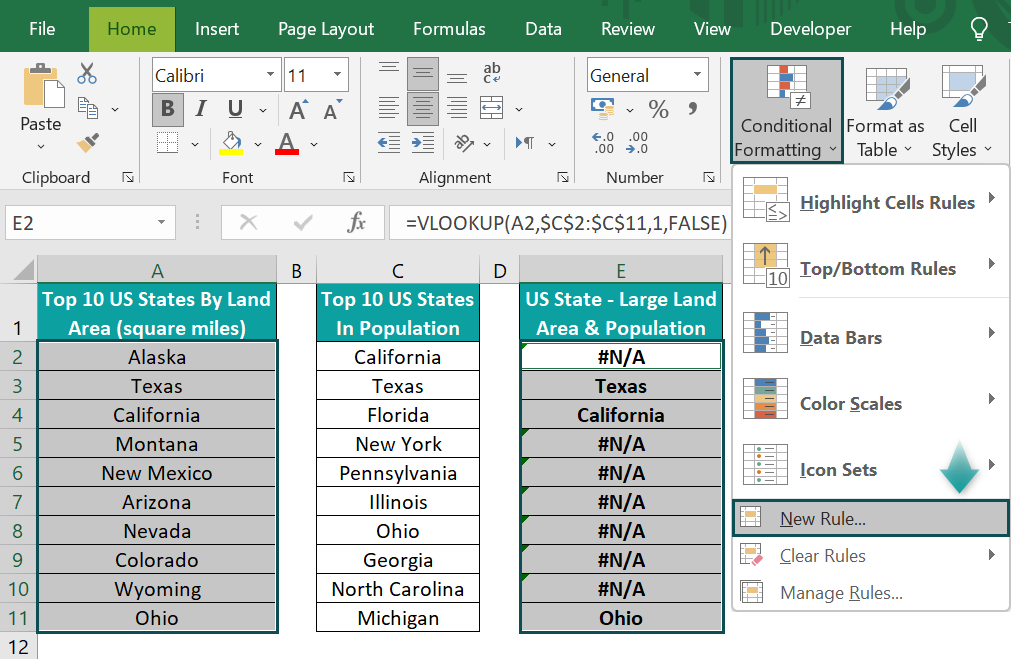The New Formatting Rule window will open.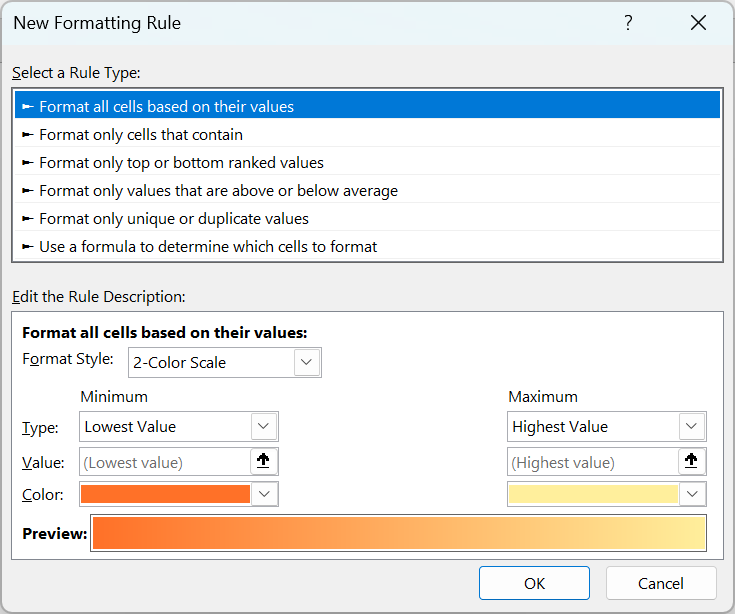And choose the last rule type to enter the conditional formatting formula in the field inside the Edit the Rule Description section.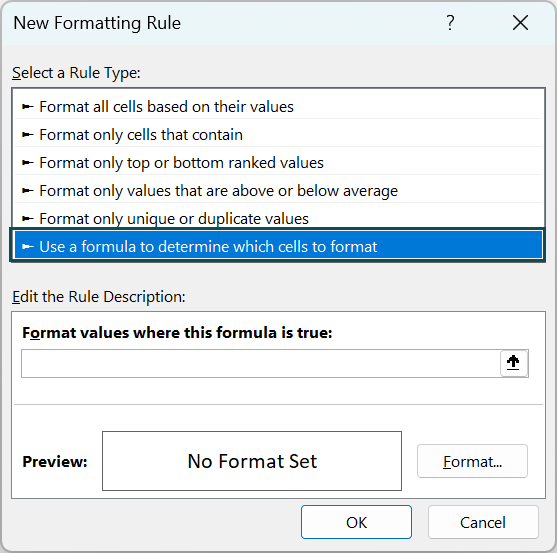5. Enter the conditional formatting formula, the VLOOKUP() within the ISERROR Excel function, in the field inside the Edit the Rule Description section.

=ISERROR(VLOOKUP(\$A2,\$C\$2:\$C\$11,1,0))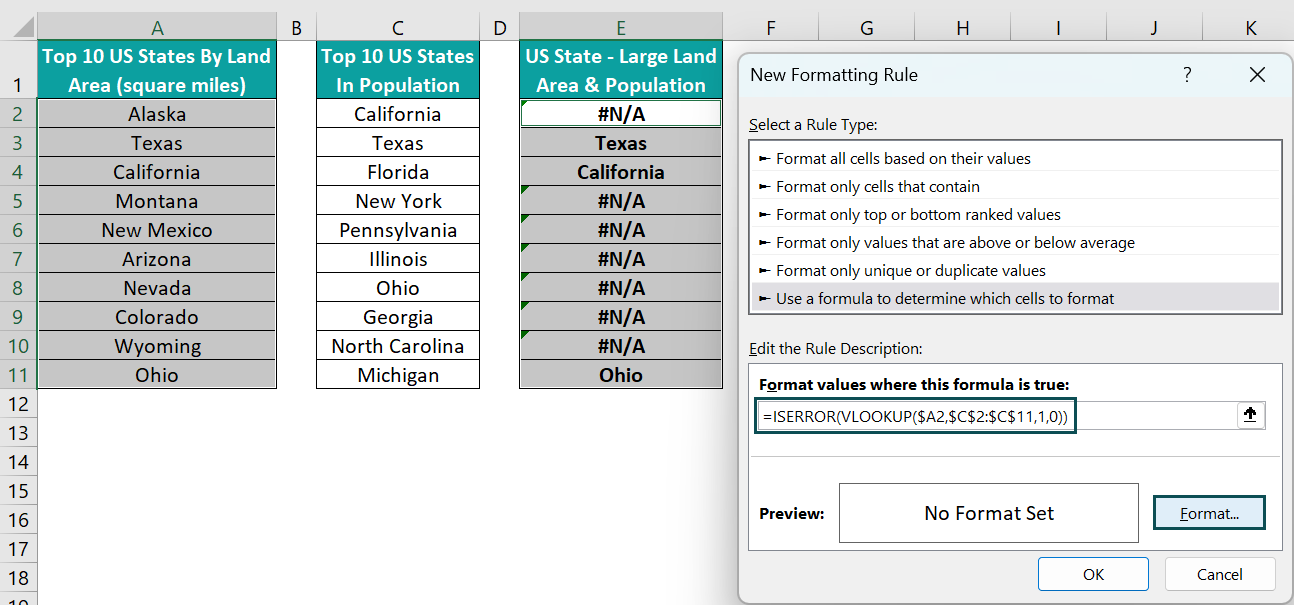And click Format to access the Excel Format Cells window.

6. Click the Fill tab to open it and choose the required background color in the Format Cells window.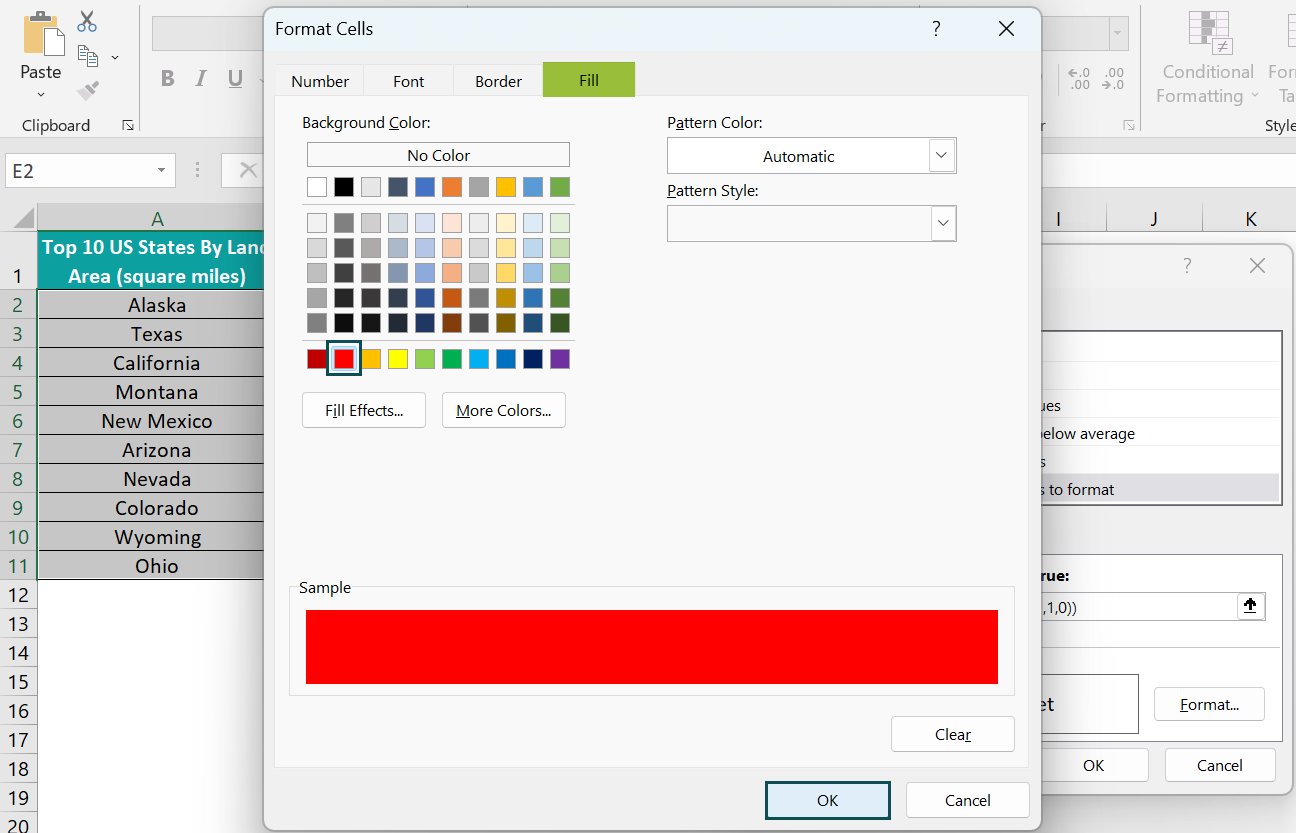And click OK in the Format Cells window.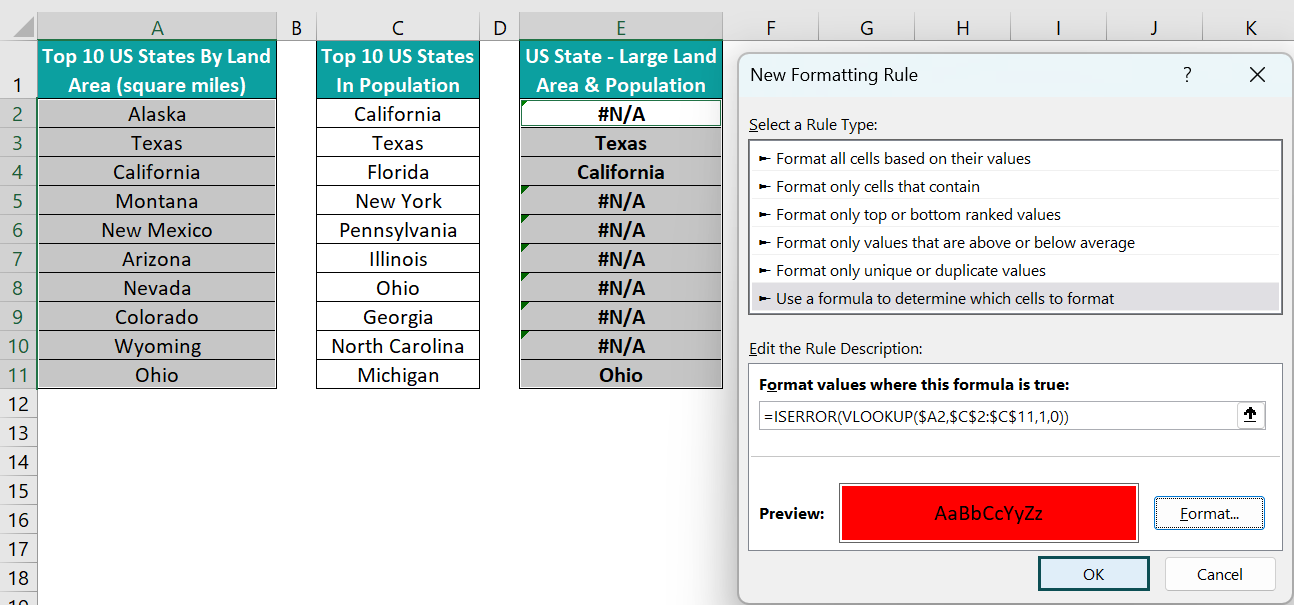And then click OK in the New Formatting Rule window.

Thus, the final output will appear as shown below.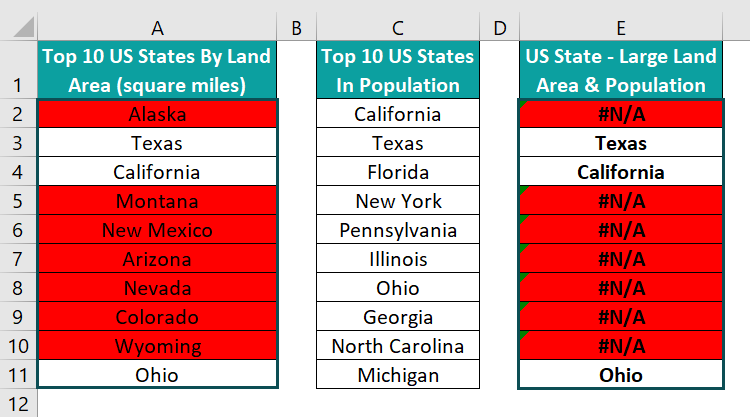First, the VLOOKUP() in the conditional formatting rule searches for a match for the column A cell lookup value in column C. But, if it does not find a match for the lookup value, it returns an error value.
Next, the ISERROR() checks if the VLOOKUP() output is an error value. And if the condition holds in a row, the corresponding chosen cells in the row get highlighted in the specified format.
The highlighted cells in column A represent the differences between columns A and C when we compare them. Likewise, the highlighted cells in column E have the same interpretation.

### Partial Lookup Using Wildcard Characters

Sometimes the lookup value may be a part of a value in the lookup range. Otherwise, the values in the lookup range may contain additional characters, even spaces, which may not be in the lookup value.

In such a case, we can perform a partial lookup using Excel Wildcard characters, ‘*’ and ‘?’. While ‘*’ represents multiple characters, ‘?’ represents one character.

We can add a ‘*’ once or ‘?‘, with the count equaling the number of characters we wish to represent using the character before and or after the lookup value in the VLOOKUP(). And we must use the Ampersand symbol to add the Wildcard characters to the lookup value in the VLOOKUP().

Let us see an example for compare two columns in Excel using VLOOKUP in different sheet with a Wildcard characters-based partial lookup.

The first two images show two columns in two worksheets.

And the requirement is to compare the two given columns to check which company names and stocks match in the two lists and display the output in column C of the second sheet.

Then, we can compare two columns in Excel using VLOOKUP in different sheet technique. And we shall perform a partial lookup of the tech company names in the stocks listed in the first sheet, using the Wildcard character, ‘*’, to get the least possible errors.

• Step 1: Choose cell C2 in the second sheet and enter the VLOOKUP() as shown below.

=VLOOKUP(“*”&A2&”*”,

Next, as the lookup range is in the first sheet, we will click the first sheet tab.

=VLOOKUP(“*”&A2&”*”,’Best Tech Stocks’!

Next, enter the absolute reference to the lookup range A2:A8, as the lookup range is constant in all the target cells, and we will copy the formula in them.

=VLOOKUP(“*”&A2&”*”,’Best Tech Stocks’!\$A\$2:\$A\$8,

Finally, we shall supply the last two argument values in the VLOOKUP() and close the bracket.

=VLOOKUP(“*”&A2&”*”,’Best Tech Stocks’!\$A\$2:\$A\$8,1,0)

• Step 2: Press Enter to view the VLOOKUP() output in cell C2.
• Step 3: Enter the formula in the remaining target cells using the fill handle.

The VLOOKUP() output in cell C7 is an error value. And the reason is that the best tech stocks list in the first sheet does not contain even a VLOOKUP partial match for the corresponding company name.

Otherwise, the partial lookup using the Wildcard character ‘*’ ensures the VLOOKUP() returns error-free output in the remaining cells.

We will check the cell C9 formula to understand how the Wildcard characters work.

First, the VLOOKUP() accepts the lookup_value as “*”&A9&”*”. The Wildcard ‘*’, before and after the excel cell reference A9, indicates the function to check the lookup range for a value containing the cell A9 value. And the value in the lookup range may or may not have additional characters before or after it.

And cell A7 in the lookup range A2:A8 in the first sheet contains the lookup value, the company name TENCENT followed by additional characters. So, Excel returns the cell A7 value from the first sheet as the output in the target cell C9.

Furthermore, had we not used the Wildcard character, the function output in all the cells would be the #N/A error. The reason is that all the company names are part of the stock names, with the stock names having additional characters. And the ‘*’ helped represent the additional characters in the stocks list, which resulted in the function partially matching the lookup value in the lookup range in each target cell.

### Important Things To Note

• When we compare two columns in Excel using VLOOKUP, the table_array rangewill only have one column. And hence, the col_index_num argument value will be 1.
• When comparing two Excel columns using the VLOOKUP() with approximate match (range_lookup argument value is TRUE), sort the column values in the table_array. Otherwise, the function may return an incorrect or error value.
• Use the Wildcard character ‘?’ and ‘*’ in the lookup_value argument to represent single and multiple characters in a string, respectively.
• The two columns comparison using the VLOOKUP function in Excel is case and format insensitive.

1. Why compare two columns in Excel using VLOOKUP is not picking up values?

The compare two columns in Excel using VLOOKUP is not picking up values, perhaps because of the following reasons:

The cells referenced contain hidden spaces or non-printable characters.
The cells referenced contain invalid data.
The lookup value is not in the first column of the lookup range.
The lookup value is smaller than the least value in the lookup range.
When setting the last argument, range_lookup, as TRUE, you did not sort the lookup range for an approximate match.

2. Can we compare more than two columns using VLOOKUP in Excel?

We can compare more than two columns using VLOOKUP in Excel using the following steps:
1) Create a helper column containing unique identifiers, developed using the lookup values from each row of the required columns. And the helper column should be the first column in the table_array.
2) Please select the first cell in the target column, where we must compare more than two columns. And enter the VLOOKUP().
The lookup_value should be created using the lookup values from the source columns, with the format as in the helper column. And the table_array range should be from the helper column up to the column, from where we must fetch the value corresponding to the lookup value in the same row as the match.
Finally, the col_index_num will be the count of the column from the helper column, from where we must fetch the value corresponding to the lookup value. And the last argument, range_lookup, will be FALSE for an exact match.
3) Press Enter to execute the VLOOKUP().

So, using the lookup values from multiple columns and the lookup range containing the helper column and one or more columns, the function compares more than two columns.

3. How do I compare 3 columns in Excel using VLOOKUP?

You can compare 3 columns in Excel using VLOOKUP by applying the method, which is explained below with an example.

The first table lists fruits, their grades and grade-based costs per carton.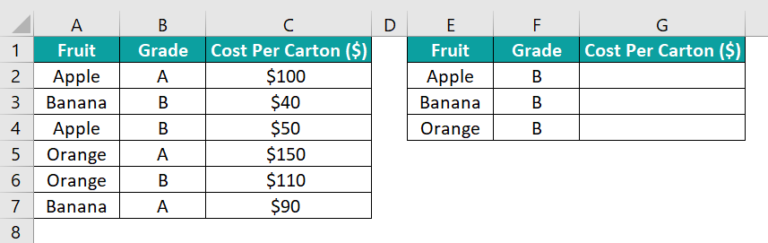And the requirement is to update the cost per carton data for the fruits and their grades mentioned in columns E and F in the second table.
So, the logic is to compare columns E, F, and C using the VLOOKUP() in the target cells to achieve the required outcome.

• Step 1: Right-click on the column C header to choose Insert from the contextual menu.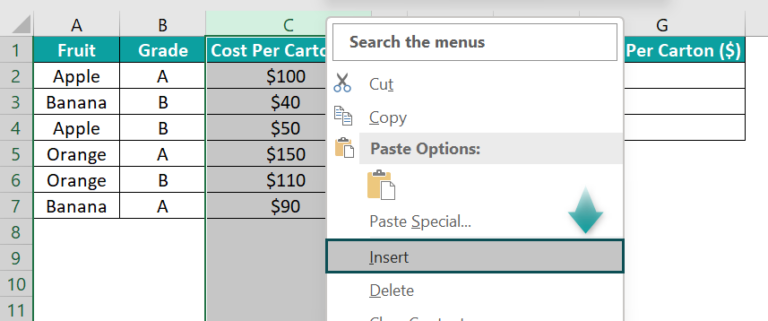The above action will insert a column before the original column C.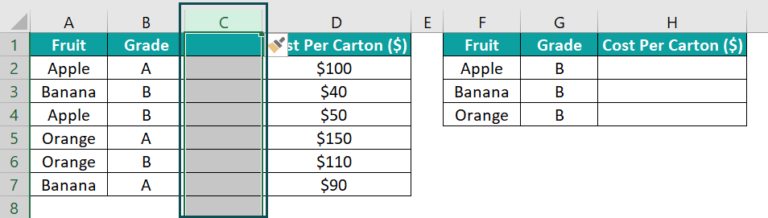Next, choose cell C1 and enter the column heading Helper.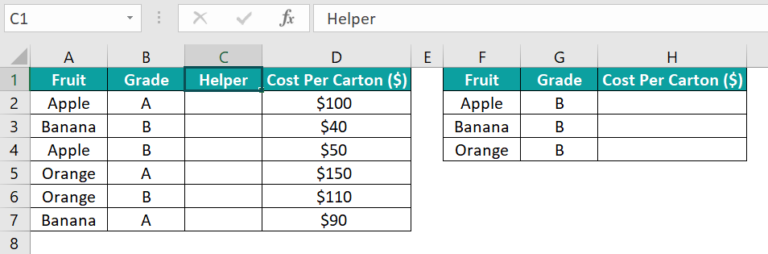• Step 2: Select cell C2 and enter the below formula.
=A2&”_”&B2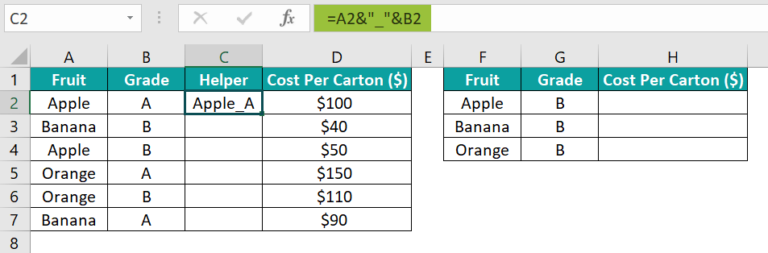Next, using the fill handle, update the formula in cells C3:C7 to create unique identifiers for the lookup values to use in the VLOOKUP() in the target cells.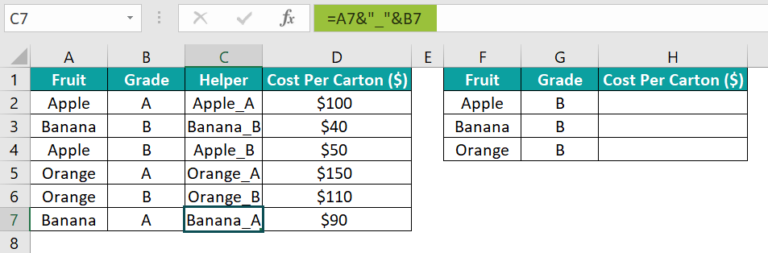• Step 3: Select the target cell H2, enter the VLOOKUP(), and press Enter.
=VLOOKUP(F2&”_”&G2,\$C\$2:\$D\$7,2,0)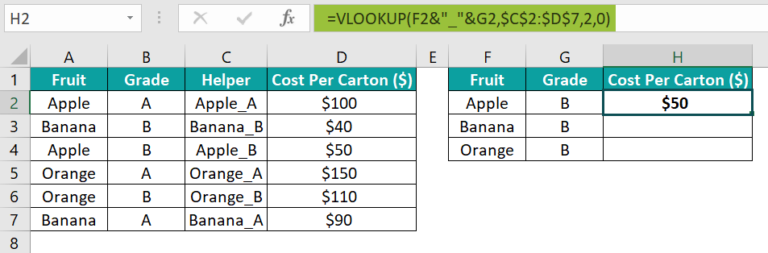• Step 4: Enter the formula in the remaining target cells using the fill handle to complete the comparison of three columns using the VLOOKUP().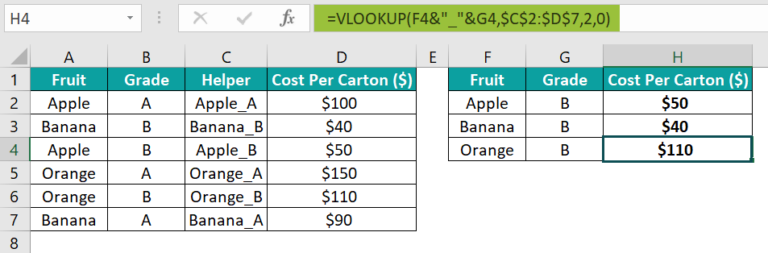The expression used for the first argument in the VLOOKUP() creates the lookup value in the same format as the entries in the Helper column. And as the Helper column is the first column in the table_array, the function searches for a match for the lookup value in the Helper column. And then, it returns the value corresponding to the lookup value in the same row as the match from column D.

Thus, columns E and F form a part of the lookup value, and column D (previously column C) is part of the lookup range. And hence, in this way, the VLOOKUP() helps compare three columns.